# 用树莓派实现实时的人脸检测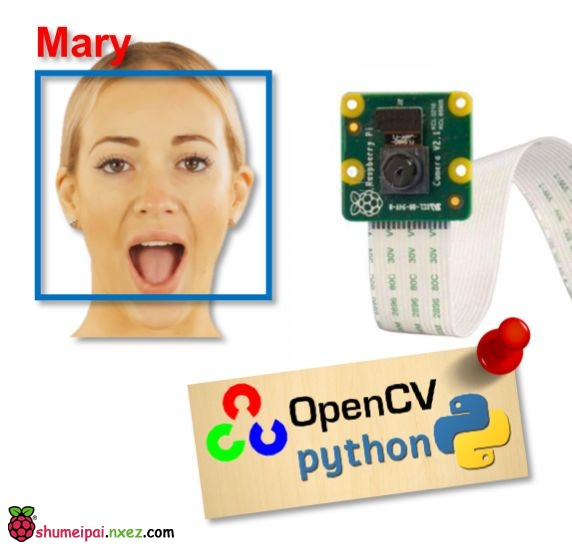#### 项目所需设备

OpenCV
Python 31）人脸检测和数据收集；
2）训练识别器；
3）人脸识别。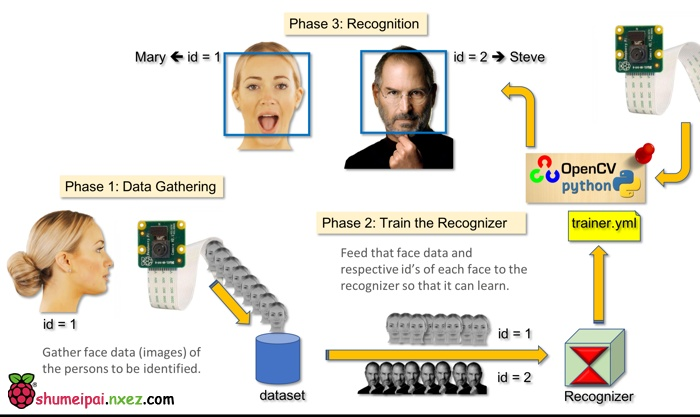#### 第1步：材料清单

500 万像素 1080p 传感器 OV5647 迷你摄像头模块

#### 第2步：安装OpenCV 3包

`source ~/.profile`

`workon cv`

`(cv) pi@raspberry:~\$`

Adrian 希望大家注意 cv Python 虚拟环境是完全独立的，且与 Raspbian Stretch 中包含的默认 Python 版本彼此隔绝。因此，全局站点包目录中的任意 Python 包对于 cv 虚拟环境而言都是不可用的。类似地，cv 站点包中的任意 Python 包对于全局 Python 包安装也都是不可用的。

`python`

`import cv2`

`cv2.__version__`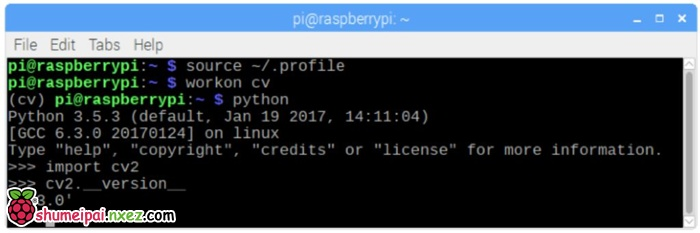上面的终端截图显示了以上步骤。

#### 第3步：测试摄像头

```import numpy as np
import cv2

cap = cv2.VideoCapture(0)
cap.set(3,640) # set Width
cap.set(4,480) # set Height

while(True):
frame = cv2.flip(frame, -1) # Flip camera vertically
gray = cv2.cvtColor(frame, cv2.COLOR_BGR2GRAY)

cv2.imshow('frame', frame)
cv2.imshow('gray', gray)

k = cv2.waitKey(30) & 0xff
if k == 27: # press 'ESC' to quit
break

cap.release()
cv2.destroyAllWindows()
```

`python simpleCamTest.py`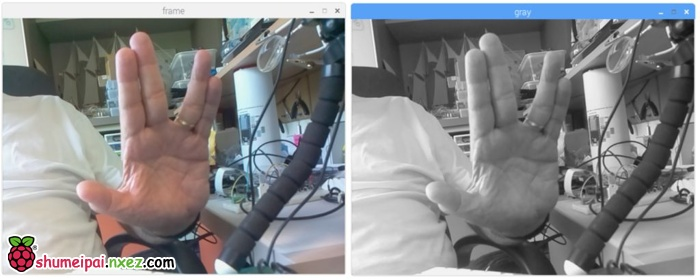#### 第4步：人脸检测

```import numpy as np
import cv2

cap = cv2.VideoCapture(0)
cap.set(3,640) # set Width
cap.set(4,480) # set Height

while True:
img = cv2.flip(img, -1)
gray = cv2.cvtColor(img, cv2.COLOR_BGR2GRAY)
gray,
scaleFactor=1.2,
minNeighbors=5,
minSize=(20, 20)
)

for (x,y,w,h) in faces:
cv2.rectangle(img,(x,y),(x+w,y+h),(255,0,0),2)
roi_gray = gray[y:y+h, x:x+w]
roi_color = img[y:y+h, x:x+w]

cv2.imshow('video',img)

k = cv2.waitKey(30) & 0xff
if k == 27: # press 'ESC' to quit
break

cap.release()
cv2.destroyAllWindows()
```

`faceCascade = cv2.CascadeClassifier('Cascades/haarcascade_frontalface_default.xml')`

```faces = faceCascade.detectMultiScale(
gray,
scaleFactor=1.2,
minNeighbors=5,
minSize=(20, 20)
)
```

gray 表示输入 grayscale 图像。
scaleFactor 表示每个图像缩减的比例大小。
minNeighbors 表示每个备选矩形框具备的邻近数量。数字越大，假正类越少。
minSize 表示人脸识别的最小矩形大小。

```for (x,y,w,h) in faces:
cv2.rectangle(img,(x,y),(x+w,y+h),(255,0,0),2)
roi_gray = gray[y:y+h, x:x+w]
roi_color = img[y:y+h, x:x+w]
```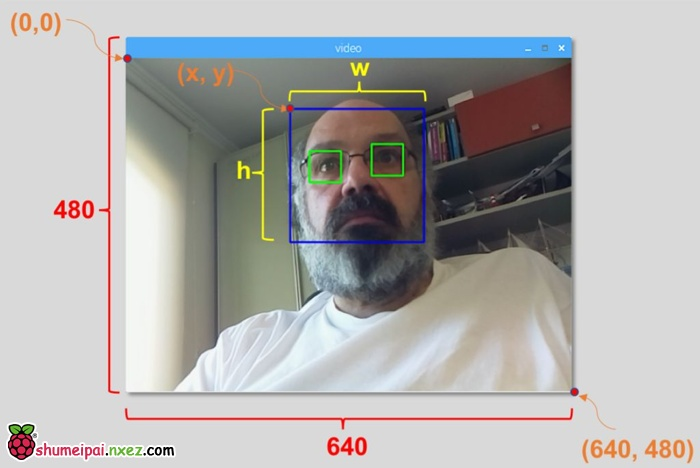`python faceDetection.py`

faceEyeDetection.py
faceSmileDetection.py
faceSmileEyeDetection.py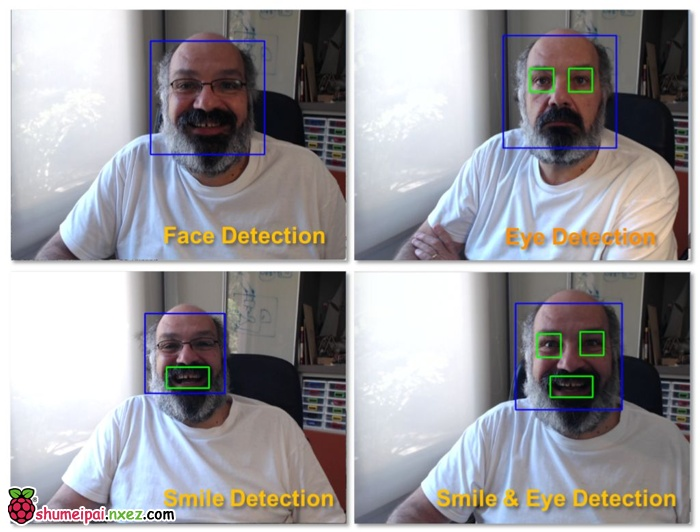#### 第5步：收集数据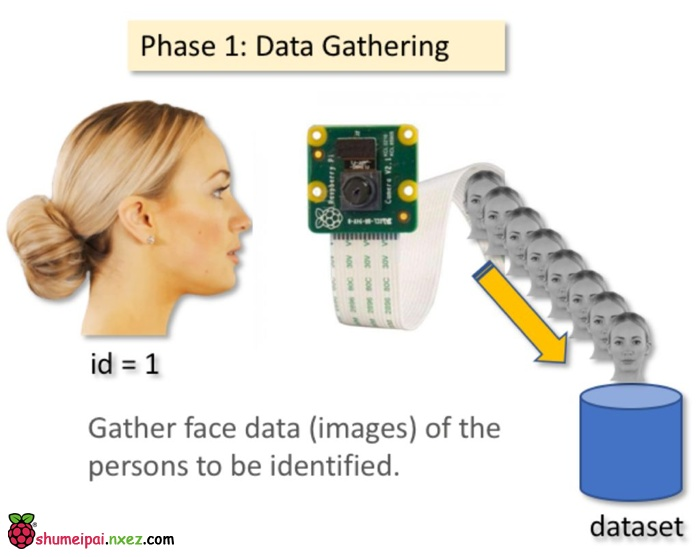`mkdir FacialRecognitionProject`

`mkdir dataset`

```import cv2
import os

cam = cv2.VideoCapture(0)
cam.set(3, 640) # set video width
cam.set(4, 480) # set video height

# For each person, enter one numeric face id
face_id = input('\n enter user id end press <return> ==>  ')

print("\n [INFO] Initializing face capture. Look the camera and wait ...")
# Initialize individual sampling face count
count = 0

while(True):
img = cv2.flip(img, -1) # flip video image vertically
gray = cv2.cvtColor(img, cv2.COLOR_BGR2GRAY)
faces = face_detector.detectMultiScale(gray, 1.3, 5)

for (x,y,w,h) in faces:
cv2.rectangle(img, (x,y), (x+w,y+h), (255,0,0), 2)
count += 1

# Save the captured image into the datasets folder
cv2.imwrite("dataset/User." + str(face_id) + '.' + str(count) + ".jpg", gray[y:y+h,x:x+w])

cv2.imshow('image', img)

k = cv2.waitKey(100) & 0xff # Press 'ESC' for exiting video
if k == 27:
break
elif count >= 30: # Take 30 face sample and stop video
break

# Do a bit of cleanup
print("\n [INFO] Exiting Program and cleanup stuff")
cam.release()
cv2.destroyAllWindows()
```

`face_id = input('\n enter user id end press  ==>  ')`

`cv2.imwrite("dataset/User." + str(face_id) + '.' + str(count) + ".jpg", gray[y:y+h,x:x+w])`

`User.face_id.count.jpg`

`User.1.4.jpg`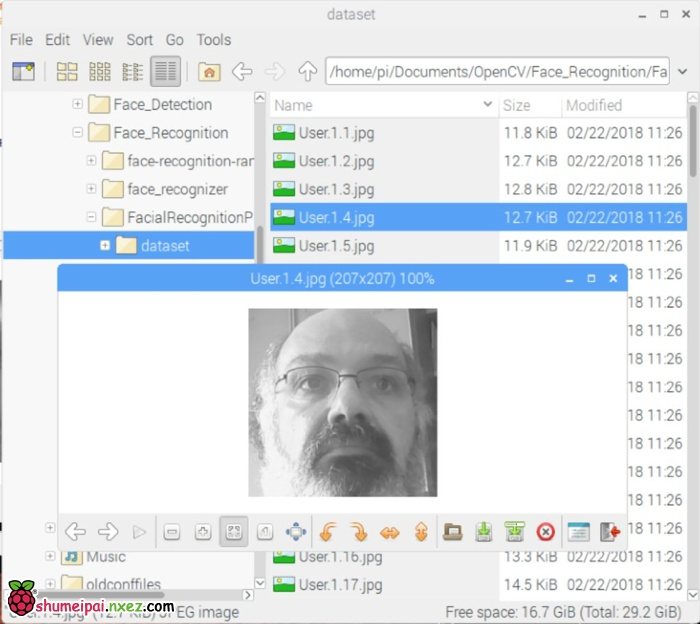#### 第六步：训练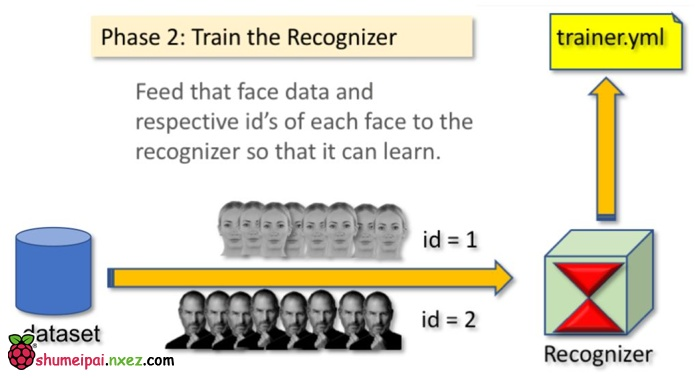`mkdir trainer`

```import numpy as np
from PIL import Image
import os

# Path for face image database
path = 'dataset'

recognizer = cv2.face.LBPHFaceRecognizer_create()

# function to get the images and label data
def getImagesAndLabels(path):
imagePaths = [os.path.join(path,f) for f in os.listdir(path)]
faceSamples=[]
ids = []
for imagePath in imagePaths:
PIL_img = Image.open(imagePath).convert('L') # convert it to grayscale
img_numpy = np.array(PIL_img,'uint8')
id = int(os.path.split(imagePath)[-1].split("."))
faces = detector.detectMultiScale(img_numpy)
for (x,y,w,h) in faces:
faceSamples.append(img_numpy[y:y+h,x:x+w])
ids.append(id)
return faceSamples,ids

print ("\n [INFO] Training faces. It will take a few seconds. Wait ...")
faces,ids = getImagesAndLabels(path)
recognizer.train(faces, np.array(ids))

# Save the model into trainer/trainer.yml
recognizer.write('trainer/trainer.yml') # recognizer.save() worked on Mac, but not on Pi

# Print the numer of faces trained and end program
print("\n [INFO] {0} faces trained. Exiting Program".format(len(np.unique(ids))))
```

`pip install pillow`

`recognizer = cv2.face.LBPHFaceRecognizer_create()`

`recognizer.train(faces, ids)`

#### 第7步：识别器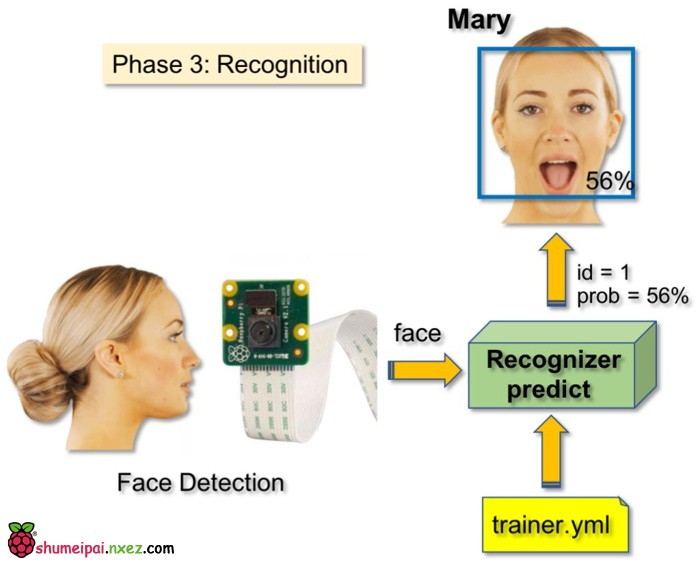```import cv2
import numpy as np
import os

recognizer = cv2.face.LBPHFaceRecognizer_create()

font = cv2.FONT_HERSHEY_SIMPLEX

#iniciate id counter
id = 0

# names related to ids: example ==> Marcelo: id=1,  etc
names = ['None', 'Marcelo', 'Paula', 'Ilza', 'Z', 'W']

# Initialize and start realtime video capture
cam = cv2.VideoCapture(0)
cam.set(3, 640) # set video widht
cam.set(4, 480) # set video height

# Define min window size to be recognized as a face
minW = 0.1*cam.get(3)
minH = 0.1*cam.get(4)

while True:
img = cv2.flip(img, -1) # Flip vertically
gray = cv2.cvtColor(img,cv2.COLOR_BGR2GRAY)

gray,
scaleFactor = 1.2,
minNeighbors = 5,
minSize = (int(minW), int(minH)),
)

for(x,y,w,h) in faces:
cv2.rectangle(img, (x,y), (x+w,y+h), (0,255,0), 2)
id, confidence = recognizer.predict(gray[y:y+h,x:x+w])

# Check if confidence is less them 100 ==> "0" is perfect match
if (confidence < 100):
id = names[id]
confidence = "  {0}%".format(round(100 - confidence))
else:
id = "unknown"
confidence = "  {0}%".format(round(100 - confidence))

cv2.putText(img, str(id), (x+5,y-5), font, 1, (255,255,255), 2)
cv2.putText(img, str(confidence), (x+5,y+h-5), font, 1, (255,255,0), 1)

cv2.imshow('camera',img)

k = cv2.waitKey(10) & 0xff # Press 'ESC' for exiting video
if k == 27:
break

# Do a bit of cleanup
print("\n [INFO] Exiting Program and cleanup stuff")
cam.release()
cv2.destroyAllWindows()
```

`names = ['None', 'Marcelo', 'Paula', 'Ilza', 'Z', 'W']`

`id, confidence = recognizer.predict(gray portion of the face)`

recognizer.predict () 将把待分析人脸的已捕捉部分作为一个参数，并返回其可能的所有者，指示其 id 以及识别器与这一匹配相关的置信度。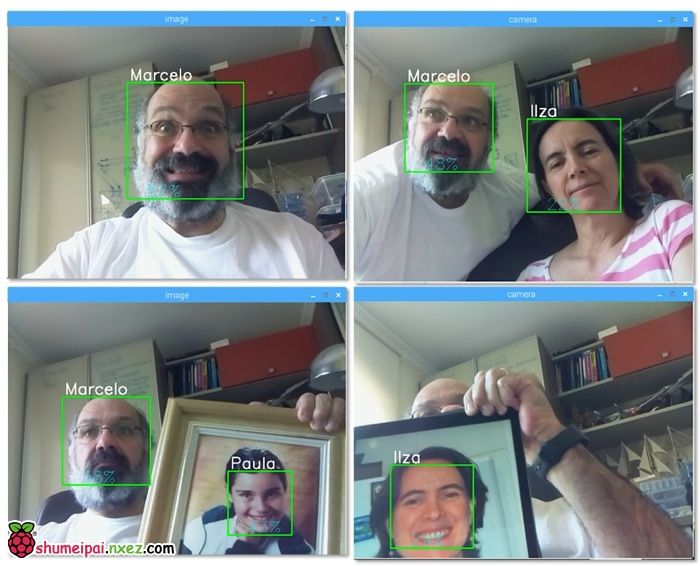#### 第 8 步：结语

（原文来自hackster，由机器之心编译）#### 33 评论

1. 大神，跪求微信号啊，我现在想做毕业设计，有不懂的地方想请教您，跪谢！！！

• 你的微信号是多少啊

2. 加油！

3. 听说是我美女老师的师兄写的文章，好厉害，好厉害

4. 这个算法是怎样的，厉害

• 计算图像位置和边距 一点点计算 没那么复杂 就是麻烦

5. 6666

6. 听说是我美女老师的师兄写的文章

7. 学习了，大神，写的好详细

8. 我想请问一下，如果想开机自动启动虚拟环境，我想到的办法是编写脚本
#！/bin/bash
source ~/.profile
workon cv
为什么workon在终端可以执行，在脚本语言中，就执行不了。帮忙解答一下，大佬

9. 你好，我也需要做一个类似的东西。对你的文章非常感兴趣。
你这个摄像头应该是那个500W像素的吧。麻烦问一下，拍照和识别的话，每秒大概多少帧啊？

谢谢！

10. 你好，在recognizer = cv2.face.LBPHFaceRecognizer_create()这一句时报错：AtrributeError:’cv2′ has no attribute ‘face’,请问为何cv2没有face，是我的环境配置有问题吗？

• 你好你这个问题解决了吗？我也出现了这个问题

• 改成recognizer = cv2.face.createLBPHFaceRecognizer()就好了

• 您好 我遇到了 AttributeError: module ‘cv2’ has no attribute ‘face’
这样一个错误，重新做了好多次了，每次都这样是环境的问题么？ 有知道的朋友 能相互交流一下么。我微信 kuyuehu

你这个问题解决了么

• ecognizer = cv2.face.LBPHFaceRecognizer_create()这一句时报错：AtrributeError:’cv2′ has no attribute ‘face’,请问为何cv2没有face，请问解决了吗

11. OpenCV Error: Assertion failed (scn == 3 || scn == 4) in cvtColor, file /home/pi/opencv-3.3.0/modules/imgproc/src/color.cpp, line 10638
Traceback (most recent call last):
File “simpleCamTest3.py”, line 9, in
gray = cv2.cvtColor(frame, cv2.COLOR_BGR2GRAY)
cv2.error: /home/pi/opencv-3.3.0/modules/imgproc/src/color.cpp:10638: error: (-215) scn == 3 || scn == 4 in function cvtColor

can you help ? error when run the sample script

• ni xian zai bian yi hao le ma? wo xian zai you tong yang de wen ti,neng gao su yi xia wo zen mo jie jue de ma?xiexie!

• 应该是Picamera没开通

12. 我想问下，用kinect1摄像头可以实现吗

13. 写的很好，step by step

14. 用树莓派3B+跑起来画面很卡，CPU占用83%，不知道有什么优化的方法

15. 详细

16. 有没有用CPP写的？

17. 你好，我运行faceDetection.py的代码，只有摄像头的影像，没有检测(蓝框)，是怎么回事?